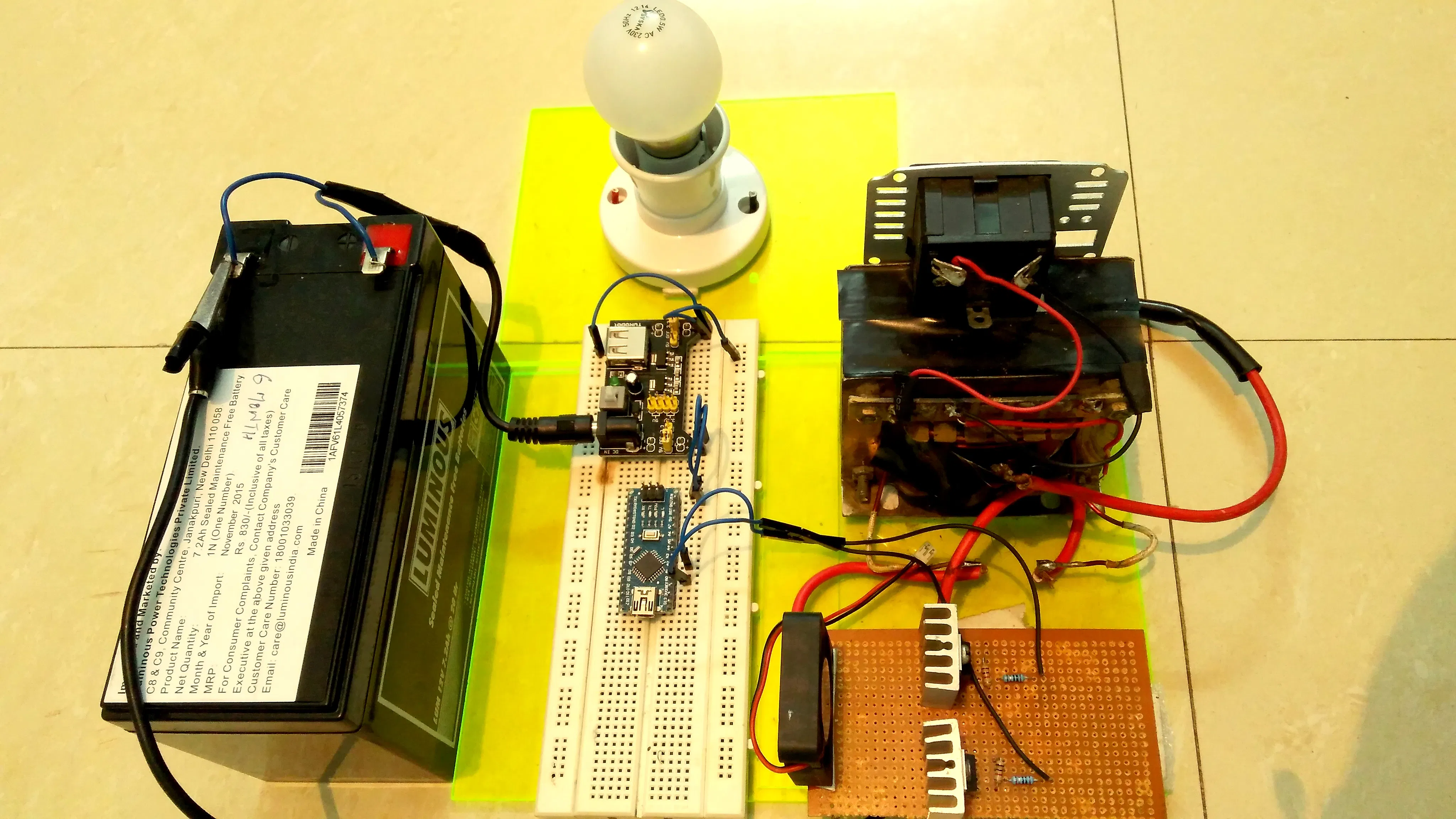Project showcaseStep by step approach is followed so that any hobbyist or design engineer can have a better understanding of the basic concepts.

• 52,007 views
• 27 respects

## Necessary tools and machines

 MultimeterSoldering iron (generic)
 Wire
 Veroboard

This project is all about designing an inverter from scratch, I am always fantasized by the projects which involves a software controlling an hardware.  With this inverter, you can power up various electronic Appliances like TV, Fan etc.

### Working:

The aim of the inverter circuit is to convert 12VDC to 220VAC, Now to achieve this, we have to first convert 12VDC to 12VAC first followed by 12VAC to 220VAC using a step up transformer.

In short, we can classify the designing of inverter circuit into three stages:

1) Driver stage

2) Power stage

3) Transformer

### Driver stage:

The tasks that are performed in driver stage are generation of modified sine wave, monitoring the battery voltage, handling the other housekeeping tasks such as short circuit protection, etc. Here I have used an Arduino NANO to accomplish all these tasks.

Arduino is generating a modified sine waveform of 5V which is amplified to a level of 12V using L293D ic. Battery voltage is monitored every 20ms using timer interrupt.

### Power Stage:

As the inverter output power is 600VA and it is 75% efficient so the input current at full load comes out to be 75Amp approx. (using ohm's law).

Now this current amplification task is performed by the power stage. In this stage, two N-Mosfets are configured in push-pull topology to amplify the current. Mosfet Chosen are IRF3205.

### Transformer:

Now this is very simple, the output waveform from the push-pull topology is fed into the transformer to generate the 220V.

Below is the video in which I have explained everything in detail.

## Code

##### Inverter arduino codeArduino
Complete code of the driver stage.
```#include "TimerOne.h"               // include TimerOne.h
#define low_battery_voltage 10.2               // define high battery voltage limit as 14.2
#define high_battery_voltage 14.4               // define low battery voltage limit as 10.2
int dutycycle = 0;               // Initailize duty cylce variable as integer data type
int sense_value =0;               // Initialize sense_value variable to capture the adc reading of battery voltage (range from 0 to 1023)
float battery_voltage = 0.0;               // Initialize battery_voltage variable as a float data type to convert sense_value to actual battery voltage

void setup()               // Setup function
{
pinMode (9,OUTPUT);              // set pin 9 as an output pin for pwm
pinMode (10,OUTPUT);             // set pin 10 as an output pin for pwm
Timer1.initialize(20000);             // Initailize timer1 time period as 20 milli second (50 Hz frequency)
Timer1.attachInterrupt(battery_voltage_measurement);      // battery_voltage_measurement function will be executed every 20 milli second using timer 1 overflow interrupt
TCCR1A = (TCCR1A & 0x0F) | 0xB0 ;             // set pin 10 inverted of pin 9
}

void battery_voltage_measurement()               // battery_voltage_measurement function starts
{ sense_value = (analogRead(A0));               // read battery voltage on pin A0 & capture that value in sense_value variable
// {warning - arduino pin accept only upto 5v so don't forget to map upper
//  battery volatge i.e 14.2v to 5v using voltage divider resistor network}

battery_voltage = sense_value * (14.4/1023.0);               // convert sense_value (0 to 1023) to range (0 to 14.2)
if(battery_voltage < 14.4 && battery_voltage > 10.2) // if battery voltage is under limit i.e between 10.2 and 14.2 then dutycycle will be 150
{
dutycycle = 300;
}

else if(battery_voltage < 10.2 || battery_voltage > 14.4)              // if battery voltage is below 10.2v or above 14.2v ,
{
dutycycle = 0;         //set the duty cycle to 0 and inverter will go in cutoff mode
}

}              // battery_voltage_measurement function ends

void loop()              // loop function starts
{
Timer1.pwm(9,dutycycle,20000);              // Timer1.pwm function takes argument as (pin no. , dutycycle , time period)
Timer1.pwm(10,1023-dutycycle,20000);

}               // loop function ends

```

## Schematics

It's complete schematic of the power inverter, drawn in EAGLE software
Inverter%20schematics.sch

#### Make an Inverter at Home Using Arduino

Project tutorial by Team Shailza

• 6,863 views
• 12 respects

#### Programmable Pocket Power Supply with OLED Display

Project tutorial by Shahariar

• 13,378 views
• 47 respects

#### Take Control Over Lego Power Functions

Project tutorial by Patrick Müller

• 86,096 views
• 87 respects

#### DIY Programmable (SCPI) Bench Power Supply

Project showcase by prasimix

• 24,068 views
• 75 respects

#### Wi-Fi Power Toggler

Project tutorial by Khalid Abdulla

• 4,115 views